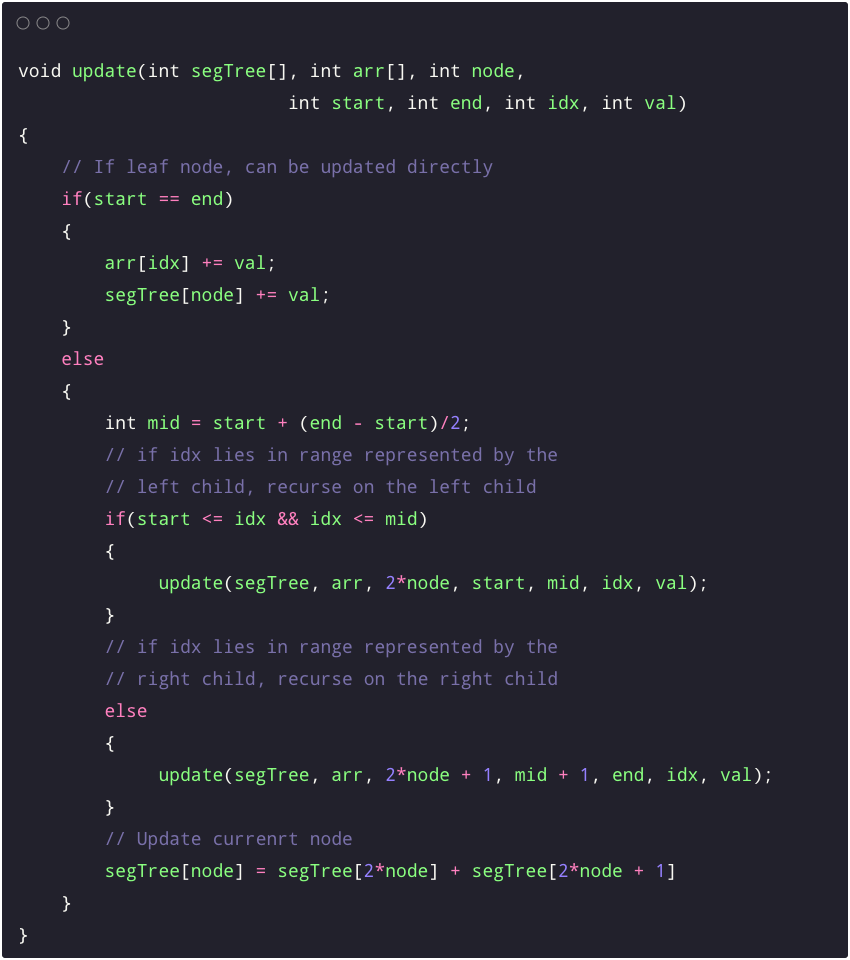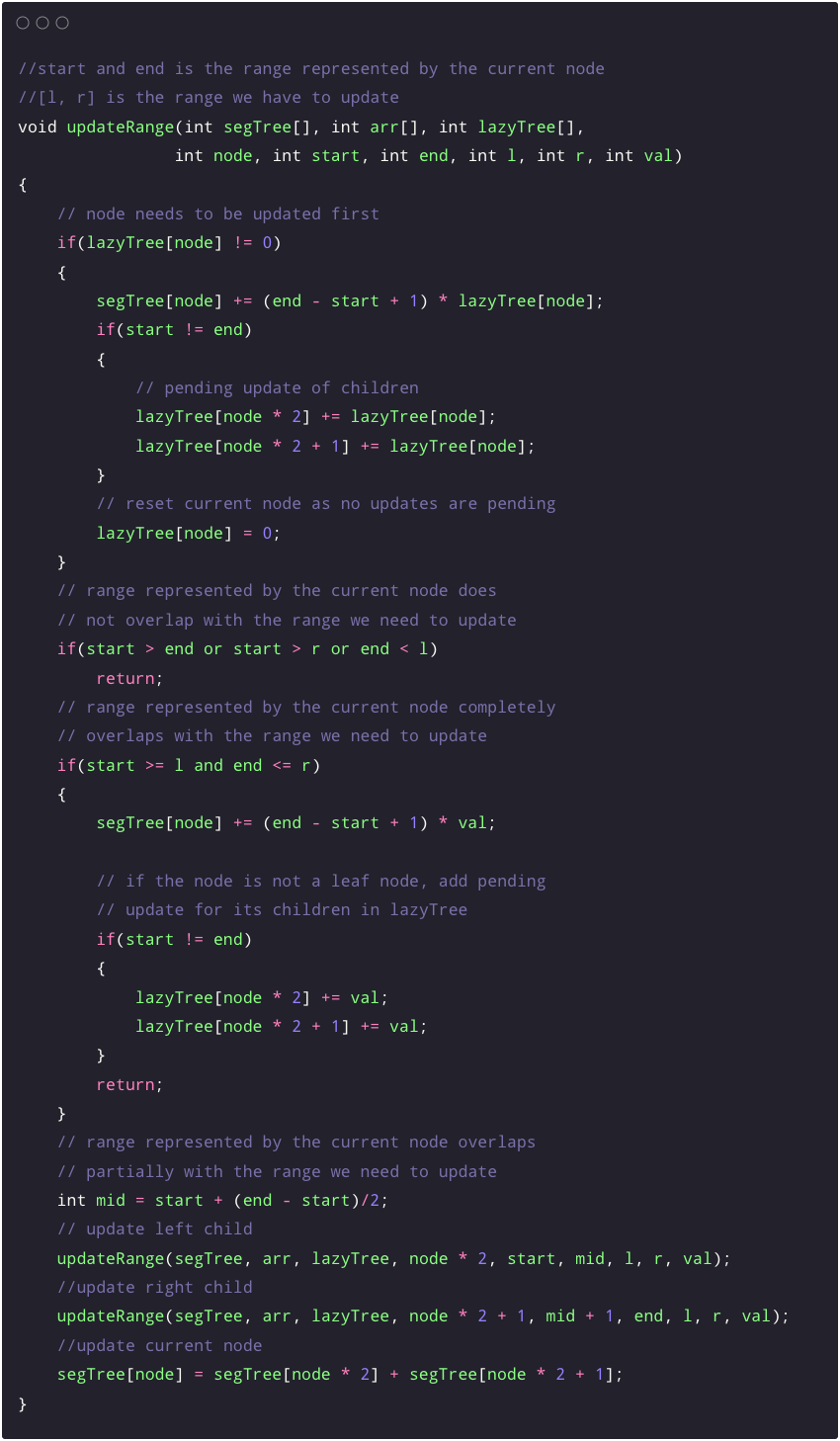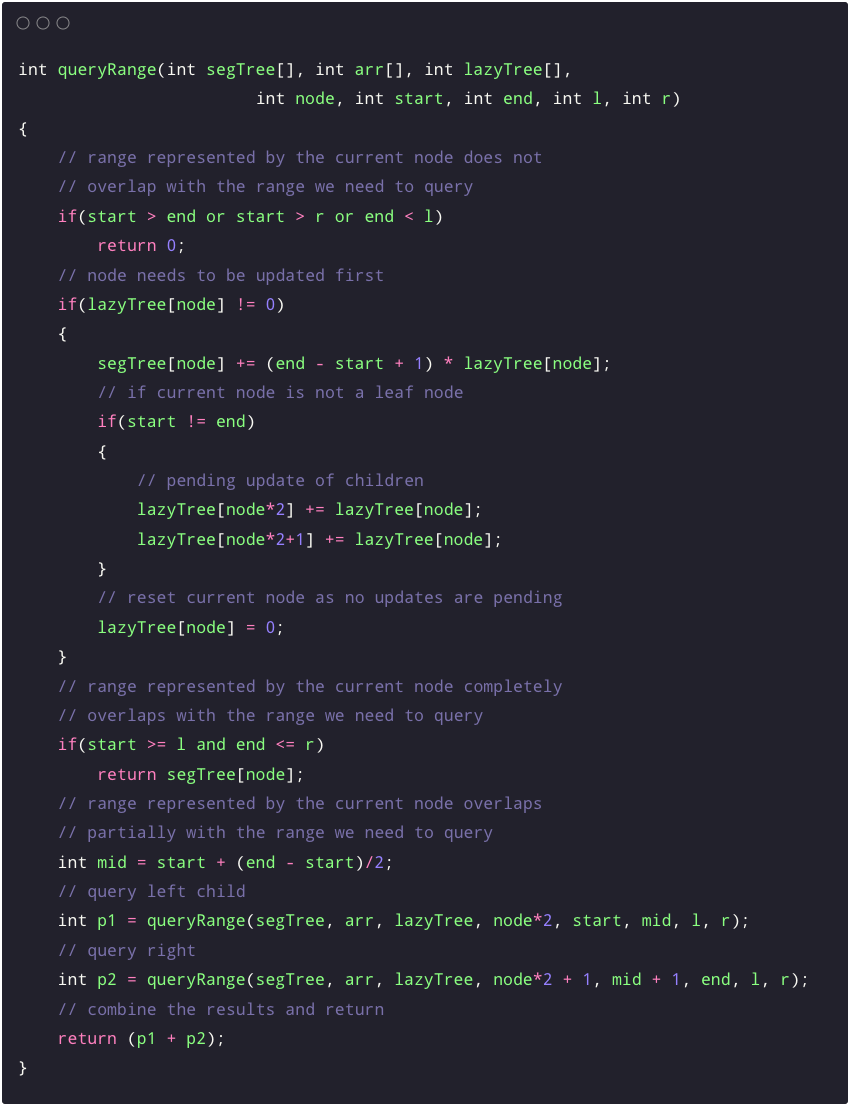# Segment Tree Part 2 (Point and Range Update, Lazy Propagation)

In the previous blog, we discussed the construction of a segment tree and range query operations on an array. Now we want to update either a single element or a range of the original array. In this blog, we will learn how to use segment trees for efficient point and range updates.

• For the point update query, update(idx, val), we need to increment the element at the index idx from the original array by val, i.e. arr[idx] = arr[idx] + val.
• For the range update query, update(l, r, val), we need to increment all the elements from index l to r from the original array by val.

## Point Updates in Segment Trees

The brute force method to do point updates in the prefix sum method discussed in the previous blog is to update that location in the array and reconstruct the prefix sum array. This method takes O(n) time and is not efficient. We will develop an O(logn) method for point updates using segment trees.

Recall that we learned how to build a segment tree in the last blog. We had seen that each node in a segment tree represents a range from the array. The leaf nodes of the segment tree represent a single index.

So a point update should first update the appropriate leaf node from the segment tree. Then we can take this update towards the root level by level by updating the parent nodes. As each level divides the segment of the array into two parts, there can be only one node in each level that needs to be updated. Thus O(logn) number of nodes needs to be updated at max.

We can implement the update function recursively. For this, we pass the root of the tree to the function at the start. The function recursively calls itself with one of the two child nodes (the one that contains the node to be updated in its range) and then recomputes its sum value, similar to the build method of the segment tree.

We define a function update(int segTree[], int arr[], int node, int start, int end, int idx, int val) that takes these values as input parameters:

• segTree[]: segment tree array
• arr[]: input array
• node: index of the current node in the segment tree
• start, end: start and end indices of the nodes represented by the current node
• idx: the index from the array which has to be updated
• val: value by which the element has to be incremented## Range Update in Segment Trees

Sometimes problems require updating an interval from l to r instead of a single element. Segment Tree allows applying modification queries to an entire segment of contiguous elements in O(logn) time.

In the brute force approach, we update all the elements in the range and construct the prefix sum array again, taking O(n) time. One more solution using segment tree point update is to update all the elements one by one. The complexity of this approach will be O(n) per range update operation, and updating a single element takes O(logn) time. Thus, the overall complexity becomes O(nlogn), worse than the prefix sum array approach.

### Lazy Propagation in Segment trees

As we know, a node in the segment tree stores a query’s value for a range of indexes. If a node range lies within the range of the update operation, then we need to update all descendants of that node. So, to improve on our last approach, we become lazy, i.e. we will do work only when required. When there is a need to update an interval, we will update the node in the segment tree, mark child nodes that require an update and update it when needed.

With the Lazy propagation idea, we update the node and postpone updates to its children by storing this update information in a separate array lazyTree[] with the same size as the segment tree. All the elements inside this array are initially zero, representing that we do not have any updates pending on node i in the segment tree. Similarly, a non-zero value of lazyTree[i] represents that this much value needs to be updated to node i in the segment tree before making any query operation.

Following are the things that we need to consider before making a range update in the segment tree:

1. If a node has any update pending, then first make that pending update to the current node.
2. If we reach a node whose range entirely lies inside the range, we need to update the current node and the lazyTree[] for the child nodes. This is the lazy approach as we do the minimum possible work to get the correct answer, and we worry about updating the remaining nodes affected by this update query only when required.
3. If the range we need to update overlaps with the range represented by the current node, we update the node similar to the point update function.

We define a function updateRange(int segTree[], int arr[], int node, int start, int end, int l, int r, int val) that takes these values as input parameters:

• segTree[]: segment tree array
• arr[]: input array
• lazyTree[]: lazy tree array that stores information about pending updates, initialized to zero initially
• node: index of the current node in the segment tree
• start, end: start and end indices of the nodes represented by the current node
• l, r: left and right indices from the array which have to be updated
• val: value by which each element from the range has to be incrementedNow, we have to change our query operation accordingly to account for the updates pending in the lazyTree array. We need to update the appropriate nodes during the query operation whose updates are pending in the lazyTree. We need to check if there is any pending update for this node. If yes, we update it and set the value in the lazyTree to zero. Then we work on finding the answer to the query, same as before.### The time complexity of lazy propagation

The time complexity of lazy propagation is O(logn). Similar to the query operation, we claim that two nodes in each level are explored further at most. The proof is discussed in the previous blog.

We will keep updating this blog with more insights later. Please share your feedback or insights. Enjoy learning, Enjoy algorithms!

☆ 16-Week Live DSA Course
☆ 10-Week Live DSA Course